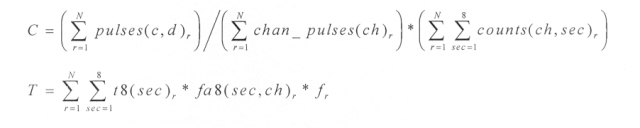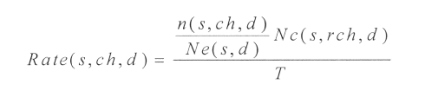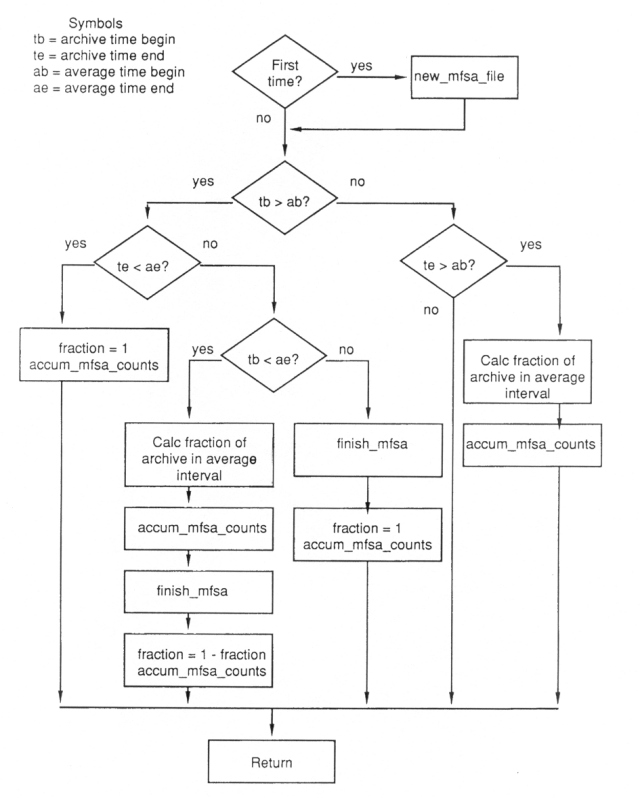ULYSSES

Ulysses HISCALE Data Analysis Handbook

## Appendix 16. LAN PHA Documentation (continued)

### A16.4 Processing (continued)

The processing for the pulse data set involves accumulations of PHA events, channel times, and total channel counts, normalizing the counts over the pulse average interval and writing the resultant count rates to the pulse rate output file.

The pulse data set is composed of count rates for each pixel in the 256 x 256 pixel space of the CA experiment. Total accumulation time for the average interval is also included to enable counts to be derived from the rates. The pulse rate data is normalized in a manner similar to the track data. The high duty cycle count rate data provides the actual ion population counts, and the low duty cycle CA event data provide the detailed composition and energy information.

Each pixel rate in the pulse rate data set is associated with a unique channel ch, a unique deposited energy pulse c, and a unique delta energy pulse d. The rates are of the form:

Rate(c,d) = C/T whereand

• r is record number.
• N is the number of records contained (completely or partially) in the current pulse average interval.
• pulses(c,d) is the count of events with deposited energy pulse=c and delta energy pulse=d in the current pulse average interval.
• chan_pulses(ch) is the total count of PHA events analyzed by channel ch in the current pulse average interval.
• counts(ch,sec) is the total particle count for channel ch and sector sec for the current pulse average interval. See track data set description of counts(ch,sec).

The top level routine for the pulse processing is process_events (described above in the track process description), and it controls the accumulation of events and rate channel counts and times. This routine is called once per archive record. Each event will increment the appropriate accumulator in the pulses and chan_pulses matrices. No sector information is retained in the pulse accumulators. As with the track data set, note that time bracketing for the pulse average interval is performed on the event level.

The accumulation of the counts and times is performed by routine accum_channel_counts. The counts and times accumulators are the same as described in the track data set. For the pulse data set the counts and times are summed over all sectors.

At the end of a pulse average interval, prior to normalizing the pulse counts and times, all pulse accumulators are summed into a second set of accumulators. These second accumulators are used to produce another output matrix spanning four (4) times the smaller pulse average interval. The routine sum_pulses performs this summation.

The normalization of the pulse rates is performed by routine norm_pulses. This routine is called at the end of the pulse average interval and converts the accumulated counts and times into normalized rates. Any events which could not be converted to rates (e.g., invalid [c,d] event pair) will remain as counts but will be made negative.

The pulse data set is written by routine write_pulses. As with the track data set, the common LAN header is set up with snapshots of the beginning and ending average times. The logical pulse output record is written as 16 physical records to the pulse rate output file.

Routine reset_pulses resets the appropriate set of accumulators after each PHA output record is written.

A16.4.5 MFSA Processing

The processing for the MFSA data set involves accumulations of sectored MFSA 32 channel spectra and total detector counts for each of the four MFSA detectors, normalizing the spectral counts over the MFSA average interval and writing the resultant rates to the MFSA output file.

The MFSA data set is composed of rates for each sector, channel, and detector in the MFSA experiment. As with the track and pulse data sets, the MFSA data set is normalized using high duty cycle, time synchronous rate data (r8, r4 in the MFSA archive record) in combination with the low duty cycle MFSA spectra. The resultant MFSA rate data contains derived ion population rates for 32 energy channels and 8 sectors over the average interval.

The MFSA rate for sector s, spectral channel ch and detector d is derived using rate channel rch and is of the form:where

• n(s,ch,d) is the accumulated spectral counts for sector s, spectral channel ch and detector d within the current MFSA average interval.
• Ne(s,d) is the total spectral count of all spectral channels within the corresponding rate channel (rch) energy bounds for sector s and detector d within the current MFSA average interval.
• Nc(s,rch,d) is the accumulated rate channel counts for sector s, rate channel rch and detector d within the current MFSA average interval.
• T is the current MFSA average interval in seconds.

The MFSA processing is controlled by routine process_mfsa. This routine will determine whether the MFSA archive record is contained in the current MFSA average interval, calculate the fraction f of the record in the current MFSA average interval, accumulate the spectra counts, and when necessary normalize and write MFSA output records (routine finish_mfsa).

The accumulation of the spectral counts and total detector counts is performed by routine accum_mfsa_counts. The routine is called once for each MFSA archive record contained (completely or partially) in the current average interval. Input to accum_mfsa_counts is the fraction f of the record contained in the average interval.

Similar to the pulse rate processing, the MFSA processing produces records containing the user specified average interval and a second average interval equal to four (4) times the specified interval. Prior to normalization, the first set of MFSA accumulators are summed into a second set of accumulators for the longer average interval in routine sum_mfsa.

The normalization of the MFSA counts is performed by routine norm_mfsa. For each detector, sector and spectral channel in the MFSA experiment, norm_mfsa will calculate the ratio n/Ne and normalize this ratio using the accumulated total detector counts for rate channel rch.

The MFSA data set is written by routine write_mfsa. The LAN common header is setup and the MFSA rate record is written to the MFSA output file.

The appropriate MFSA accumulators are reset following the output of an MFSA record by routine reset_mfsa.

Figure A16-5 Process_mfsa flow diagram